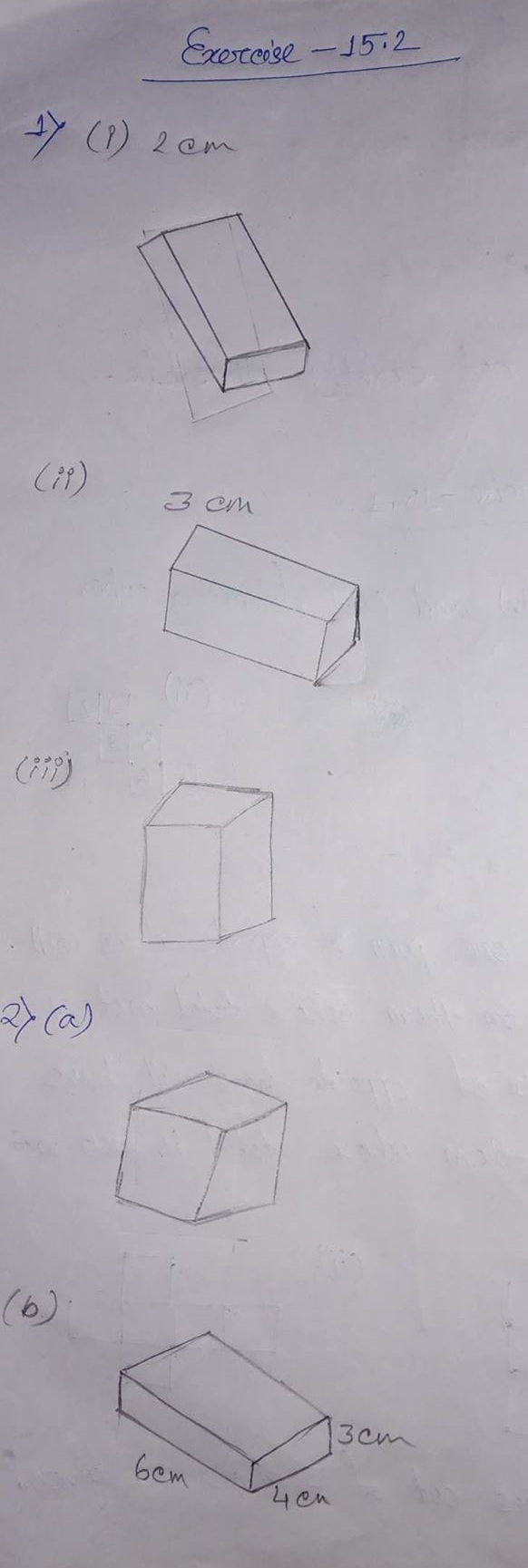# ML Aggarwal CBSE Solutions Class 7 Math Fifteenth Chapter Visualising Solid Shapes Exercise 15.2

### ML Aggarwal CBSE Solutions Class 7 Math 14th Chapter Visualising Solid Shapes Exercise 15.2

(1) The dimensions of a cuboid are 5 cm, 3 cm and 2 cm. Draw three different isometric sketches of this cuboid with height

(i) 2 cm (ii) 3 cm (iii) 5 cm

(2) Give (i) an oblique sketch (ii) an isometric sketch for each of the following:

(a) A cube with an edge 4 cm long.

(b) A cuboid of length 6 cm, breadth 4 cm and height 3 cm.

Solution:(3) The adjoining figure shows an isometric sketch of a 3D shape. Draw an oblique sketch that matches this sketch.

Solution:Updated: November 22, 2019 — 12:57 pm